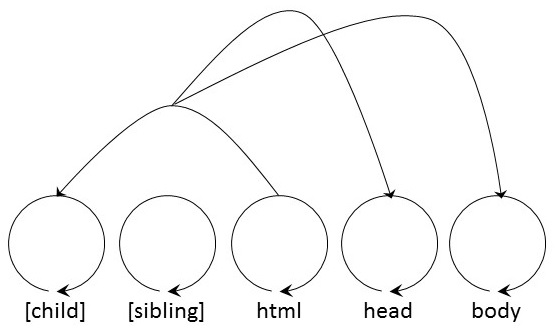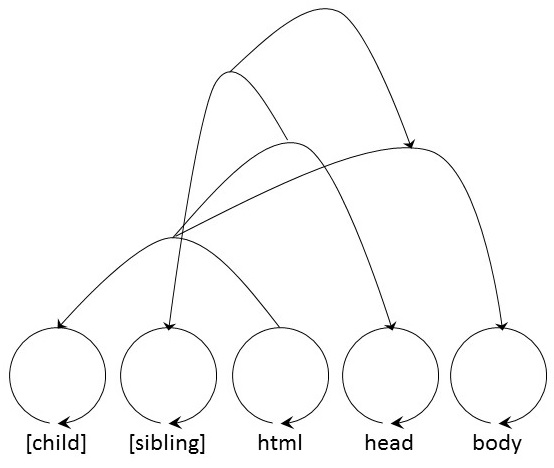# Storing HTML in a Rhizome

This post demonstrates how it is possible to use rhizomes to store simple HTML.

Consider the following HTML.

How could we store this in a rhizome? First of all, it would make sense to treat every HTML tag as an atomic symbol. There are three such symbols in the sample: html, head and body. Let us assume that, unless qualified otherwise, the direction of a relation indicates a parent-child relationship. Thus, (x, y) must be read as “x is parent of y”. The html tag has two children and they are ordered. html is both parent of head and of body, but head is the first child and body is the second. How could we express this fact? At this time, it is useful to introduce qualifiers.

Definition:

A _qualifier_ _q_ is a relation which, when paired with another relation _ri_, indicates how that relation _ri_ should be processed.

We will use square brackets `[]` to denote a relation as a qualifier.

In the next table terminal relations were assigned to each HTML tag. Additionally, two qualifiers were introduced, one for child relations and a second one for sibling relations.

HTML Tag Terminal Relation
[child] (0, 0)
[sibling] (1, 1)
html (2, 2)
body (4, 4)

It is now possible to express the HTML above as two different, subsequent relations:

1. `((html, [child]), head) = (((2, 2), (0, 0)), (3, 3))`
2. `((html, [child]), body) = (((2, 2), (0, 0)), (4, 4))`

Or expressed tree-like:

 ``````( ( html, [child] ), head ) ( ( html, [child] ), body )`````` ``````( ( (2, 2), (0, 0) ), (3, 3) ) ( ( (2, 2), (0, 0) ), (4, 4) )``````

Expressed graphically:Note that we did not add a comma `,` between the head and the body relation. The two sibling tags head and body are not directly related to each other. Adding a [sibling] qualifier, we pair the two relations and end up with:

1. `((((html, [child]), head), [sibling]), ((html, [child]), body)) = (((((2, 2), (0, 0)), (3, 3)), (1, 1)), (((2, 2), (0, 0)), (4, 4)))`

Or expressed as a tree:

 ``````( ( ( ( html, [child] ), head ), [sibling] ), ( ( html, [child] ), body ) )`````` ``````( ( ( ( (2, 2), (0, 0) ), (3, 3) ), (1, 1) ), ( ( (2, 2), (0, 0) ), (4, 4) ) )``````

This is a graphical representation of the same tree.Using only the simple rules above, we can now go on adding tags. Each tag is either a child or a sibling in relation to another one.

Imagine that two clients store the same atomic symbol table and use the same encoding algorithm. It is now possible to send a single (possibly very long) integer number over a network, and the receiver can fully re-compute the complete HTML tree. Of course, a possibility for actually storing content is still missing. For this purpose, we could introduce a further qualifier [value]. Once the atomic symbol table is complete concerning valid HTML tags, we start adding one numbered variable per plain, textual content. A relation `(si, [value])` would indicate a textual content stored in variable si. Of course it would be necessary to also submit the plain textual content of every variable over the network. The result would look something like this:

</tr> </table> with `s1 = "Hello World!", s2 = "My First Heading"`. The same procedure would be applicable to tag attributes. First, introduce a new qualifier [attribute]. Then, add all valid HTML attributes (such as id, name, href etc.) to the atomic symbol table. (Be aware that this does not prohibit us to create meaningless combinations of tags and attributes such as `<table href="">`.) The textual content variables are then stored after the tag attributes in the atomic symbol table.
HTML Tag Terminal Relation
[child] (0, 0)
[sibling] (1, 1)
html (2, 2)